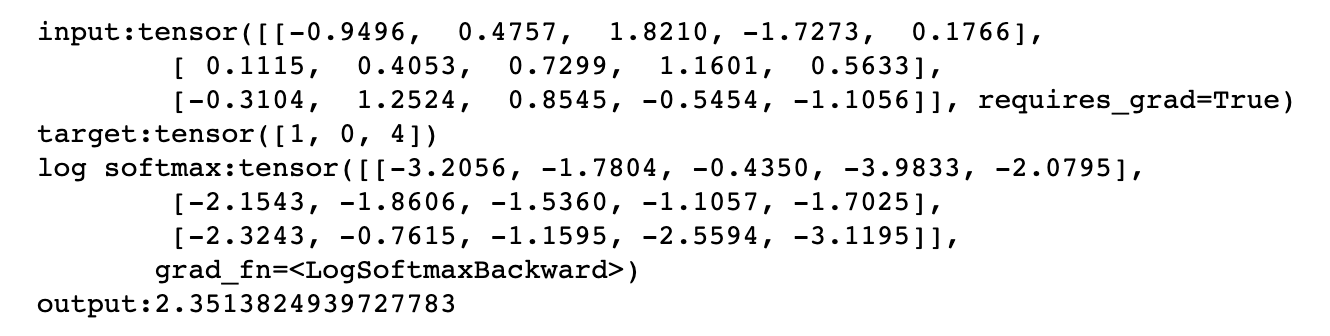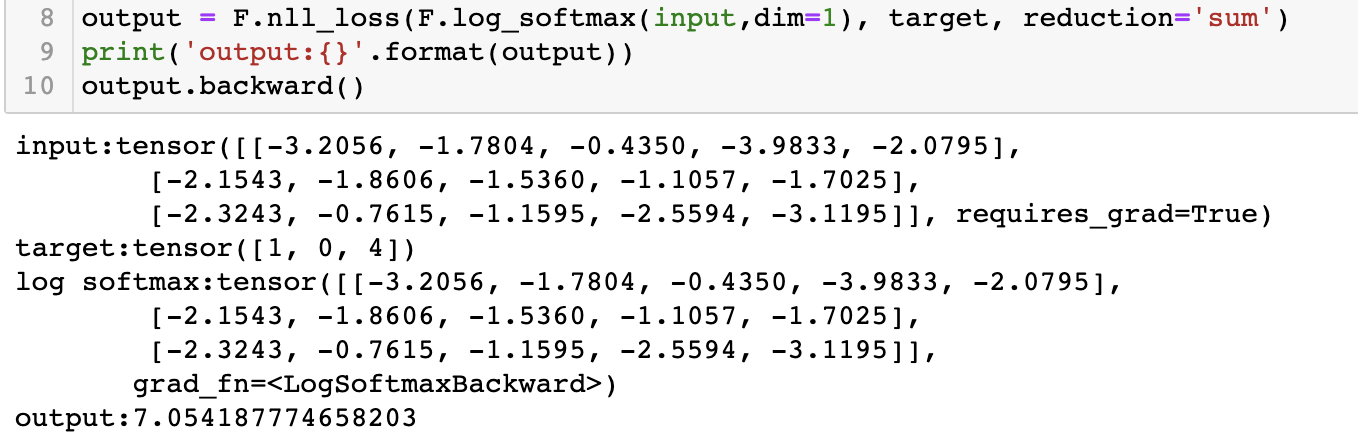# 【pytorch笔记】损失函数nll_loss

## 热门标签

,GentleCP

# 使用场景

# 训练
for batch_idx, (data, target) in enumerate(train_loader):
data, target = data.to(device), target.to(device)
output = model(data)
loss = F.nll_loss(output, target)
loss.backward()
optimizer.step()
# 测试
data, target = data.to(device), target.to(device)
output = model(data)
test_loss += F.nll_loss(output, target, reduction = 'sum')

# 函数理解

• input:(N,C),其中C表示分类的数量，N表示数据的条数，由于数据的输入是按batch输入，所以N也是batch的大小。
• target:(N)目标结果，即常见分类任务中的label，包含N个。
• reduction：对计算结果采取的操作，通常我们用sum（对N个误差结果求和）,mean（对N个误差结果取平均），默认是对所有样本求loss均值

# 例子演示

input = torch.randn(3, 5, requires_grad=True)
# each element in target has to have 0 <= value < C
target = torch.tensor([1, 0, 4])
print('input:{}\n target:{}'.format(input,target))
print('log softmax:{}'.format(F.log_softmax(input,dim=1)))
output = F.nll_loss(F.log_softmax(input,dim=1), target)
print('output:{}'.format(output))
output.backward()

jupyter notebook打印一下中间结果：• log softmax tensor(模型output):

tensor([[-3.2056, -1.7804, -0.4350, -3.9833, -2.0795],
[-2.1543, -1.8606, -1.5360, -1.1057, -1.7025],
[-2.3243, -0.7615, -1.1595, -2.5594, -3.1195]]
• target:

tensor([1, 0, 4])

标签代表了tensor中每一行向量应该检查的位置，例如第一个标签是1，这表示在tensor第一行中应该选择1号位置的元素-1.7804（代表了模型将数据分为1类的概率）取出，同理取第2行0号位置元素-2.1543,取第三行4号位置元素-3.1195，将它们去除负号求和再取均值。
则该模型输出outputtarget之间误差应为：(1.7804+2.1543+3.1195)/3 = 2.3514

• reduction
同样是上面的输入，我们添加reductionsum，查看output结果：发现计算结果是7.054...，说明没有执行前面 (1.7804+2.1543+3.1195)/3 = 2.3514求均值的操作。直接将各个样本与label之间的误差求和返回。

# 总结

nll_loss 函数接收两个tensor第一个是模型的output，第二个是label targetoutput中每一行与一个标签中每一列的元素对应，根据target的取值找出output行中对应位置元素，求和取平均值。

### 评论 抢沙发

Vieu4.5主题Q Q 登 录